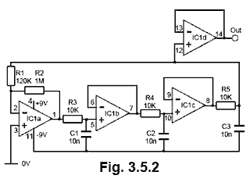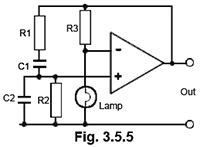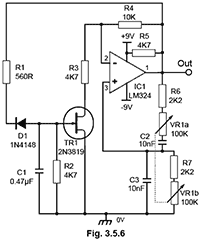# AF Oscillators Quiz

Try this quiz, based on the information you can find in Oscillators Module 3. Submit your answers and see how many you get right. If you get any answers wrong. Just follow the hints to find the right answer and learn about Oscillators as you go.

### 1.

Refer to Fig. 3.5.1. How much is the feedback signal phase shifted by C3,R4,?### 2.

Refer to Fig. 3.5.1. What is the approximate frequency of oscillation?

### 3.

An advantage of Wien Bridge oscillators compared to Phase Shift types is that::

### 4.

If each low pass filter in Fig. 3.5.2 has a voltage gain of 0.5, how much gain will be required from the amplifier for oscillations to start?### 5.

Refer to Figs. 3.5.2.and 3.5.1 Which of the following comparisons is true?

### 6.

Which formula in Fig 3.5.3 should be used for finding the frequency of oscillation of a buffered phase shift oscillator?### 7.

If the circuit in Fig. 3.5.4 is supplied with an AC signal and the ammeter reads zero amperes, what is the phase relationship between points X and Y?### 8.

What is the purpose of the lamp in Fig. 3.5.5?### 9.

Refer to Fig 3.5.6. What is the purpose of TR1?### 10.

Refer to Fig 3.5.6. What is the approximate low frequency limit of the Oscillator?

Top of Page